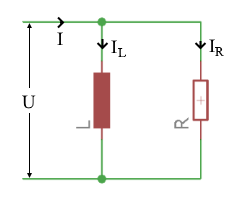# Resistor and inductor in parallel

Calculator and formulas for calculating resistor and inductor in parallel

## Calculate resistor and inductor in parallel

The computer calculates the voltages, power, current, impedance and reactance for a resistor and inductor in parallel.

RL in parallel calculator

 Input Coil H mH µH nH Resistor mΩ Ω kΩ MΩ Frequency Hz kHz MHz GHz Voltage mV V kV Decimal places 0 1 2 3 4 6 8 10 Results Resistance XL Impedance Z Current in resistor IR Current in the coilIL Current I Real power P Reactive power Q Apparent power S Phase angle φI Total current IR Current through the resistor IL Current through the coil Y Admittance [1/Z] XL Inductive reactance R Effective resistance Z Impedance G Conductance [1/R] BL Susceptance [1/XL] P Real power S Apparent power QL Inductive reactive power φ Phase shift in °

## Formulas and description for RL in parallel

The total resistance of the RL parallel circuit in AC is called impedance Z. Ohm's law applies to the entire circuit.

Current and voltage are in phase at the ohmic resistance. The inductive reactance of the capacitor lags the current the voltage by −90 °.

The total current I is the sum of the geometrically added partial currents. For this purpose, both partial flows form the legs of a right triangle. Its hypotenuse corresponds to the total current I. The resulting triangle is called the current triangle or vector diagram of the currents.

### Current triangle### Conductance triangle

When connected in parallel, the partial currents behave like the conductance values of resistances.### Resistance triangle### Power triangle### Power factorIst diese Seite hilfreich?             Vielen Dank für Ihr Feedback! Wie können wir die Seite verbessern?